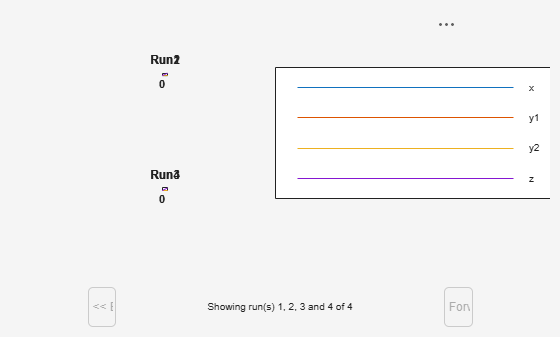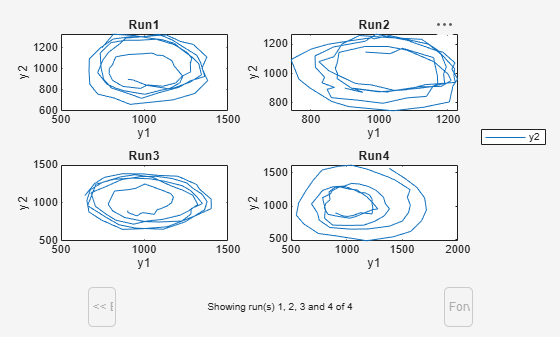Documentation

# sbiosubplot

Plot simulation results in subplots

## Syntax

``sbiosubplot(sd)``
``sbiosubplot(sd,fcnHandle,xArgs,yArgs)``
``sbiosubplot(sd,fcnHandle,xArgs,yArgs,showLegend)``
``sbiosubplot(sd,fcnHandle,xArgs,yArgs,showLegend,Name,Value)``

## Description

example

````sbiosubplot(sd)` plots each simulation run from `sd`, a `SimData` object or array of objects, into its own subplot. The subplot is a time plot of each state in `sd`.```

example

````sbiosubplot(sd,fcnHandle,xArgs,yArgs)` plots simulation results by calling the function handle `fcnHandle` with inputs `sd`, `xArgs`, and `yArgs`. The inputs `xArgs` and `yArgs` must be cell arrays of the names of the states to plot.```

example

````sbiosubplot(sd,fcnHandle,xArgs,yArgs,showLegend)` also specifies whether to show the legend in the plot. If `true`, the function shows `yArgs` as the legend.```

example

````sbiosubplot(sd,fcnHandle,xArgs,yArgs,showLegend,Name,Value)` also uses additional options specified by one or more name-value pair arguments. For example, you can specify the x-label and y-label of the plot.```

## Examples

collapse all

Plot the prey versus predator data from the stochastically simulated lotka model in separate subplots by using a custom function (`plotXY`).

Load the model. Set the solver type to SSA to perform stochastic simulations, and set the stop time to 3.

```sbioloadproject lotka; cs = getconfigset(m1); cs.SolverType = 'SSA'; cs.StopTime = 3; rng('default') % For reproducibility```

Set the number of runs and use `sbioensemblerun` for simulation.

```numRuns = 4; sd = sbioensemblerun(m1,numRuns);```

Plot each simulation run in a separate subplot. By default, `sbiosubplot` shows the time plot of each species for each run per subplot.

`sbiosubplot(sd); `Plot selected states against each other; in this case, plot the prey population versus the predator population in separate subplots for each run. Use the function `plotXY` (shown at the end of this example) to plot the simulated y1 (prey) data versus the y2 (predator). Specify the function as a function handle in the `sbiosubplot` call to plot each run in its own subplot. In this case, the fifth input argument (`showLegend`) is set to `true`, which means the fourth input argument (`yArgs`) is shown as the legend.

If you use the live script file for this example, the `plotXY` function is already included at the end of the file. Otherwise, you must define the `plotXY` function at the end of your .m or .mlx file or add it as a file on the MATLAB path.

`sbiosubplot(sd,@plotXY,{'y1'},{'y2'},true,'xlabel','y1','ylabel','y2')`Define plotXY Function

sbiosubplot accepts a function handle of a function with the signature:

`function functionName(sd,xArgs,yArgs)`.

The `plotXY` function plots two selected states against each other. The first input `sd` is the simulation data (SimBiology `SimData` object or vector of objects). In this example, `xArgs` is a cell array containing the name of the species to be plotted on the x-axis, and yArgs is a cell array containing the name of the species to be plotted on the y-axis. However, you can use the inputs xArgs and yArgs in any way in your custom plotting function. No output from the function is necessary.

```function plotXY(sd,xArgs,yArgs) % Select simulation data for each state from each run. xData = selectbyname(sd,xArgs); yData = selectbyname(sd,yArgs); % Plot the species against each other. plot(xData.Data,yData.Data); end ```

## Input Arguments

collapse all

Simulation results, specified as a `SimData` object or vector of `SimData` objects.

This argument corresponds to the first input of the function referenced by `fcnHandle`.

Example: `simdata`

Function to generate line plots, specified as a function handle. For an example of a custom function to plot selected species from simulation data, see Plot Selected States from Simulation Data in Subplots.

The function must have the signature:

`function functionName(sd,xArgs,yArgs)`.

The inputs `sd`, `xArgs`, and `yArgs` are the same inputs that you pass in when you call `sbiosubplot`. No output from the function is necessary.

Example: `@plotXY`

Data Types: `function_handle`

State names to plot, specified as a string vector or cell array of character vectors. For instance, you can use `xArgs` to represent the states to be plotted on the x-axis of your custom plot.

This argument corresponds to the second input of the function referenced by `fcnHandle`.

Example: `{'y1'}`

Data Types: `cell`

State names to plot, specified as a string vector or cell array of character vectors. For instance, you can use `yArgs` to represent the states to be plotted on the y-axis of your custom plot.

This argument corresponds to the third input of the function referenced by `fcnHandle`.

Example: `{'y2','z'}`

Data Types: `cell`

Logical flag to show the plot legend, specified as `true` or `false`. If `true`, the function shows `yArgs` as the legend.

Example: `true`

Data Types: `logical`

### Name-Value Pair Arguments

Specify optional comma-separated pairs of `Name,Value` arguments. `Name` is the argument name and `Value` is the corresponding value. `Name` must appear inside quotes. You can specify several name and value pair arguments in any order as `Name1,Value1,...,NameN,ValueN`.

Example: `'xlabel','Species A'` specifies the x-label of the plot.

Label for the x-axis of the plot, specified as the comma-separated pair consisting of `'xlabel'` and a character vector or string.

Example: `'xlabel','y1'`

Data Types: `char` | `string`

Label for the y-axis of the plot, specified as the comma-separated pair consisting of `'ylabel'` and a character vector or string.

Example: `'ylabel','y2'`

Data Types: `char` | `string`

## See Also

#### Introduced in R2008a

##### SupportGet trial now